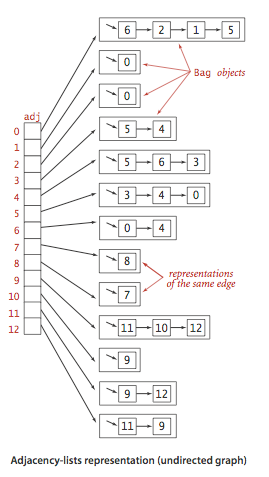# Using Javascript To Build An Undirected Graph

Now that my time at Hack Reactor is almost over, I finally am able to set aside some time to write some blog posts.

Early in the Hack Reactor program, we had a two day sprint where we implemented several data structures, but interestingly enough, we were never tasked with building a graph data structure. So, for my inaugural post I've decided to discuss the undirected graph data structure and provide my own implementation in Javascript.

A general definition for a graph is as follows:
A graph is a set of vertices and a collection of edges that each connect a pair of vertices. Specific types of graphs owe their specialization to either having their edges denote direction and or having their edges denote a specified weight. An Undirected Graph does not specify a direction or a weight on it's edges. Given this definition, we can begin to think about how we would go about creating a data structure that represents a Undirected Graph.

There are a few ways to represent an Undirected Graph as the following passage from section 4.1 of the book Algorithms (4th Edition) explains:

• An adjacency matrix, where we maintain a V-by-V boolean array, with the entry in row v and column w defined to be true if there is an edge adjacent to both vertex v and vertex w in the graph, and to be false otherwise.
• An array of edges, using an Edge class with two instance variables of type int.
• An array of adjacency lists, where we maintain a vertex-indexed array of lists of the vertices adjacent to each vertex.

I chose option 3 for my implementation with a slight modification in that I'm using an object whose keys are the vertices and the corresponding values are the adjacency lists. The figure below illustrates the array based implementation but is similar enough to my implementation to be representative.Now for the code.

Below is the Graph constructor which creates an instance of a Undirected Graph object. The property `_adjacencyLists` is be the collection of adjacency lists for each vertex (see figure above).

``````var Graph = function() {
this._numOfEdges = 0;
};
``````

An adjacency list is essentially a linked list of all of the adjacent vertices of a particular vertex. The code for the AdjacencyList constructor and it's methods is shown below.

``````var AdjacencyList = function() {
this.tail = null;
};

var vertex = new Vertex(value);

} else {
this.tail.next = vertex;
}

this.tail = vertex;
};

var detached = null;

return null;
} else {
detached.next = null;
return detached;
}
};
``````

You probably noticed in the AdjacencyList's `add` method that I am instantiated a vertex object. The code for the Vertex constructor is given below.

``````var Vertex = function(value) {
this.value = value;
this.next = null;
};
``````

Now that we have the code for all of our supporting data structures I can now introduce the remaining code for the Undirected Graph data structure.

The `addEdge` method shown below is responsible for building the graph, one edge at a time. This is accomplished by indexing into the `_adjacencyLists` collection using vertex v and either adding vertex w to the corresponding adjacency list or creating a new adjacency list and then adding vertex w.

``````Graph.prototype.addEdge = function(v, w) {
this._numOfEdges++;
};
``````

I've also included a couple of convenience methods that will allow us to display a list of vertices and their corresponding adjacency lists. The code for `getVertices` and `toString` is shown below.

``````Graph.prototype.getVertices = function() {
};

Graph.prototype.toString = function() {
var currentNode = null;
var vertices = this.getVertices();

console.log(vertices.length + " vertices, " +
this._numOfEdges + " edges");
for (var i = 0; i < vertices.length; i++) {
while (currentNode) {
adjString += " " + currentNode.value;
currentNode = currentNode.next;
}
}
};
``````

Given the following test code calling on our graph's `toString` method will produce the following:

``````var graph = new Graph();

graph.toString();

4 vertices, 4 edges
1: 2 3 4
2: 1
3: 1 4
4: 1 3
``````

So there you have it, an Undirected Graph built using Javascript. Hopefully you were able to learn a little something from this blog post. Feel free to share.#### Wayne Montague

Front-End Engineer with a passion for beautiful UI, data visualization and interaction design. Oakland, CA is the place I call home.